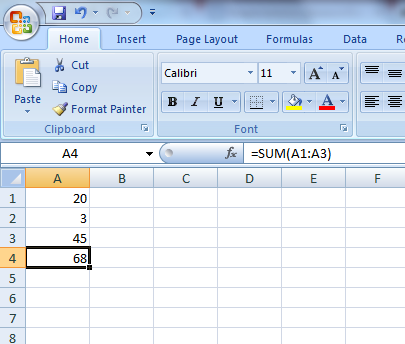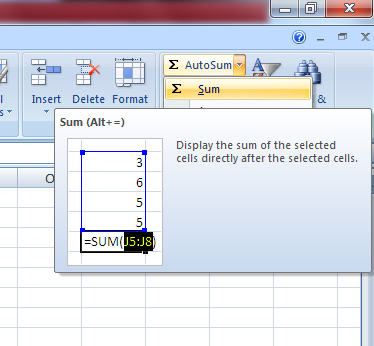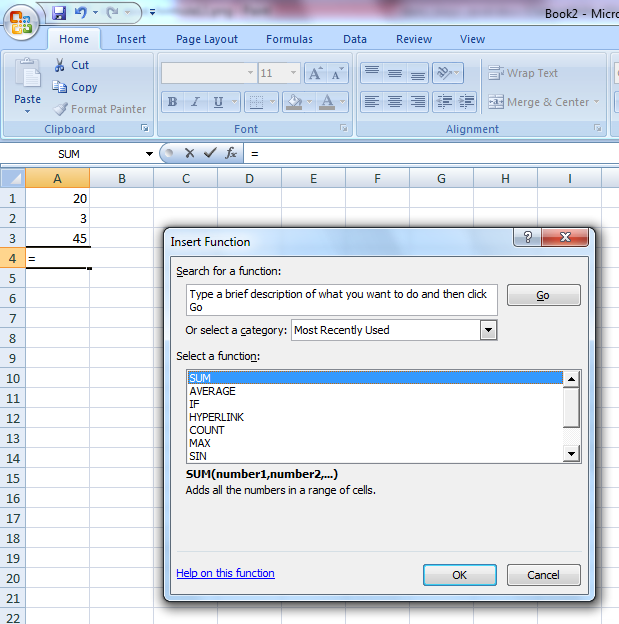# How to use functions in Excel

Function in Excel – this is a predetermined formula, which works with one or several values and returns the result.

Most of all used functions - this is a jotting of common formulae.

Let's consider an example, the function =SUM(В1:В3) – is the same as the formula =В1+В2+В3.

This is a simple function. But there are functions which make very complicated operations.

The composition of the function – this is the name and the argument. So, in the example above SUM – is the name, and В1:В3 – the argument. It is put in round parentheses

More often one can meet the function "the sum", that's why the button "AutoSum" is located on the panel of the toolbar "Home".

Fill the cells A1, A2, A3 with any numbers. Click on the cell A4, and then click on the button "AutoSum". Now press the Enter key. The formula of the sum of the cells from A1 till A3 will be input into the cell A4."AutoSum" button has a dropdown sheet. One can choose another formula from it.In order to choose another function, one should press the button “Paste function”. It is located in the formula bar. A window will appear.It's possible to use the field of search functions, if you don't know which function you need exactly.

Here there is also a dropdown sheet "Category", in which it is possible to choose the category of the function, and then to choose the function itself. It is chosen in the window "Select a function".

When making the formula of the reference, the cells can be replaced with table headers. In the settings it's set up by default that Excel doesn't read headings in formulas. In order to turn on this option, it's necessary to select "Parameters" in menu "Service". In the tab Calculations set flag Accept range names.